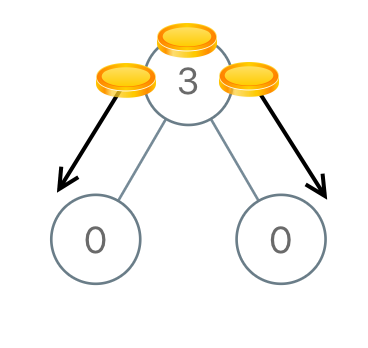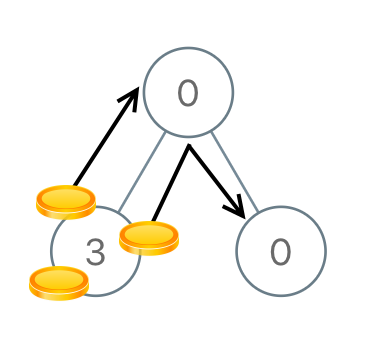# Distribute Coins in Binary Tree

## Problem

### Example 1`Input: root = [3,0,0]Output: 2Explanation: From the root of the tree, we move one coin to its left child, and one coin to its right child.`

### Example 2`Input: root = [0,3,0]Output: 3Explanation: From the left child of the root, we move two coins to the root [taking two moves]. Then, we move one coin from the root of the tree to the right child.`

### Constraints

• The number of nodes in the tree is `n`.
• `1 <= n <= 100`
• `0 <= Node.val <= n`
• The sum of all `Node.val` is `n`.

## Solution

`// move might be a negative numberabs(move)`

`func distributeCoins(root *TreeNode) int {  steps := 0  dfs(root, &steps)  return steps}`

`dfs` 函式就是整個問題求解的核心。

## Implementation

`func dfs(root *TreeNode, steps *int) int {+  if root == nil {+    return 0+  }}`

```func dfs(root *TreeNode, steps *int) int {  if root == nil {    return 0  }
+ left := dfs(root.Left, steps)+ right := dfs(root.Right, steps)
// Do something...}```

`steps = steps + abs(left) + abs(right) // 0 + 0 + 0`

`coins = root.Val - 1 + left + right // 0 - 1 + 0 + 0 = -1， -1 表示需要被分配一枚硬幣`

```// implement Do something...left := dfs(root.Left, steps) // -1，代表需要分配一枚硬幣right := dfs(root.Right, steps) // -1，代表需要分配一枚硬幣steps = steps + abs(-1) + abs(-1) // 0 + 1 + 1 = 2，代表需要移動兩次
return root.Val - 1 + left + right // 3 - 1 + (-1) + (-1) = 0```

```left := dfs(root.Left, steps) // 3 - 1 + 0 + 0 = 2right := dfs(root.Right, steps) // -1steps = steps + abs(2) + abs(-1) // 0 + 2 + 1 = 3
return root.Val - 1 + left + right // 0 - 1 + 2 + (-1) = 0```

## 完整實作

```func distributeCoins(root *TreeNode) int {  steps := 0  dfs(root, &steps)  return steps}
func dfs(root *TreeNode, steps *int) int {  if root == nil {    return 0  }
left := dfs(root.Left, steps)  right := dfs(root.Right, steps)  *steps = *steps + abs(left) + abs(right)
return root.Val - 1 + left + right}```